Detalles de la revistaFormato
Revista
eISSN
2083-831X
Primera edición
09 Nov 2012
Calendario de la edición
4 veces al año
Idiomas
Inglés
Acceso abierto

# Analysis of crack propagation in a “pull-out” test

###### Aceptado: 04 Jun 2019
Detalles de la revistaFormato
Revista
eISSN
2083-831X
Primera edición
09 Nov 2012
Calendario de la edición
4 veces al año
Idiomas
Inglés

The article describes a computer analysis of the pull-out test used to calculate the force needed to pull out a rock fragment and determine the shape of this broken fragment. The analyzed material is sandstone and porphyry. The analysis included the first approach to using own subroutine in the Simulia Abaqus system, that is, which task is undertaken to accurately determine the crack path of the Finite Element Method model. The work also contains a description of laboratory tests and analytical considerations.

#### Keywords

Introduction

The “pull-out” test is quite often used as a simple method of estimating the material parameters of concrete or rock without the need for laboratory test. The test consists of pulling out the anchor placed in the material. On the basis of the test results, tensile strength and fracture energy can be estimated. Most often, this test is performed for concrete with an anchor, which was embedded during the formation of the sample (Brencich, 2015; Wang, Wu, Ouyang, He, & Sun, 2017; Contrafatto & Cosenza, 2014). Thus, the characteristics of the pull-out test is completely different from that described in their papers. The authors of the presented work attempted to analyze sandstone cracking during a pull-out test made with a self-undercutting anchor, which only has contact with the tested material in the undercut area. These anchors are normally used to anchor various structural elements. The described test is intended for the opposite purpose – to pull out the anchor together with a part of the rock. This is a different approach, because the anchor is designed not to destroy the material in which it is mounted, but to destroy itself. The analysis focused on the sandstone obtained from the quarries Braciszów and Brenna in Poland, but there were some tests concerning the porphyry from the quarry Zalas in Poland. Rock samples were obtained during the “in situ” pull-out tests at the mentioned quarries, which were carried out as a part of the Rodest project financed by the Polish National Center of Science (Jonak et al., 2019).

The research consisted of finding the parameters of the selected material – sandstone from the Braciszów quarry. Next, the numerical analysis of the pull-out test was carried out using the Finite Element Method with the use of X-FEM elements, which allowed simulation of the crack propagation giving results not dependent on the FEM mesh. The pulling process was forced by the vertical displacement of the upper edge of the anchor. Contact rock-anchor was analyzed taking into account the different friction coefficients. The results obtained in the calculations were compared with the pull-out tests performed on actual rock. The aim of the described research is to find a way to calculate the force of pulling out the anchor for any material and for any length of anchoring.

The HILTI HDA-P M20x250/100 anchor was adopted for pull-out tests. with anchoring length of 25 cm will be used (“European Technical Assessment…”), the picture of this anchor is shown in Fig. 1.

To mount this anchor, it is placed in a prepared hole in the anchored surface. Then, a drill is attached to the anchor, and while drilling, the anchor undercuts itself with deflecting elements. Scheme of mounting the anchor is shown in Fig. 2. Fixing the anchor, therefore, consists of a contact between the material and the undercut, not the anchor side. So the contact area is relatively small.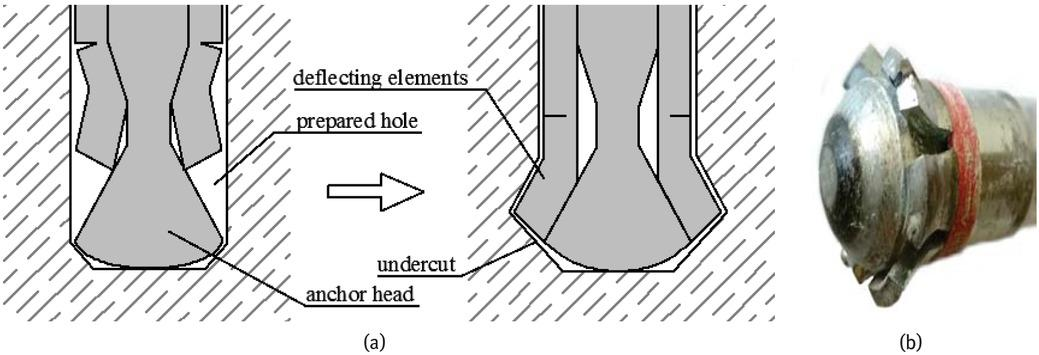a) scheme of mounting the anchor, b) used anchor.
Description of the Model

Simulia Abaqus FEA system was used for calculations. The X-FEM method implemented in Abaqus was used for modelling the crack. The pull-out test was modeled in 2D space as an axially symmetrical task. The computational

model is presented in Fig. 3, where h is the depth of the anchorage. The boundary conditions were modelled on the right and bottom edge, the size of the model was assumed to be large enough so that the boundary conditions did not affect the result.

The sandstone was modelled as a linear-elastic material with Young’s modulus E = 15.7749 GPa, Poisson’s ratio ν = 0.251. Maximum principal stress (Rankine criterion) was chosen as a parameter responsible for the material damage, the following material parameters: tensile strength ft = 12.89 MPa and the critical strain energy release rate GIc = 0.306 N/mm were used. The laboratory tests from which these parameters were obtained are described in the further subsection. Tensile strength ft is also the stress that initiates the crack.

The load was simulated by several different methods. The first method consists of modeling the action of the anchor by forcing the vertical displacement in the area of the rock undercut fragment (see Fig. 3). This method was divided into two variants. First, with horizontal blocking on the anchor edge, and second, without this blocking. Next method was with an anchor in the model. The load was simulated by vertical displacement applied on the upper edge of the anchor, and a contact was modeled between the anchor and sandstone, with 5 different friction coefficients: h = 0.01, 0.1 0.2, 0.5 and 1.0. There was no possibility to determine the exact friction coefficient between these two material because in this test, the rock under heavy load crushes, but still this crushed part transfers the load further.

The critical force was determined by the sum of the vertical reactions in nodes with applied displacement, both in the case of the load specified in the place of contact of two materials and for the load at the end of the anchor.

Material Parameters

Correct modelling in the program requires the determination of material parameters. A series of laboratory tests were performed on the samples of the selected material.

Compression test

Cubes of 7 x 7 x 7 cm dimensions were used to determine a few material parameters. They were used to calculate the Young modulus and Poisson’s ratio as a result of the compression with extensometer tests. Then, the compressive strength was obtained from the destructive compression test performed on the same samples.

Photograph from these tests are shown in Fig. 4. On the left side, the displacement sensor is visible, which measures the vertical deformations, and on the right side, there is the extensometer that measures the horizontal deformations. It is mounted on steel plates glued to the opposite sides of samples.

The Young modulus was calculated from the below equation:

$E=h⋅κA$$$E=\frac{h\cdot \kappa }{A}$$

where, h is the height of the sample, A is the area of horizontal cross-section of the sample and κ is the slope of the curve of compressive force to vertical deformations’ dependence, read in the range where the curve is close to a straight line. Results from this test are shown in Fig. 5a There were 14 cubic samples named K1–K14, but some tests were unsuccessful. The Young modulus varied from 10.136 to 24.768 GPa. Mean value was 15.745 GPa with standard deviation 4.819 GPa (31% of the mean value).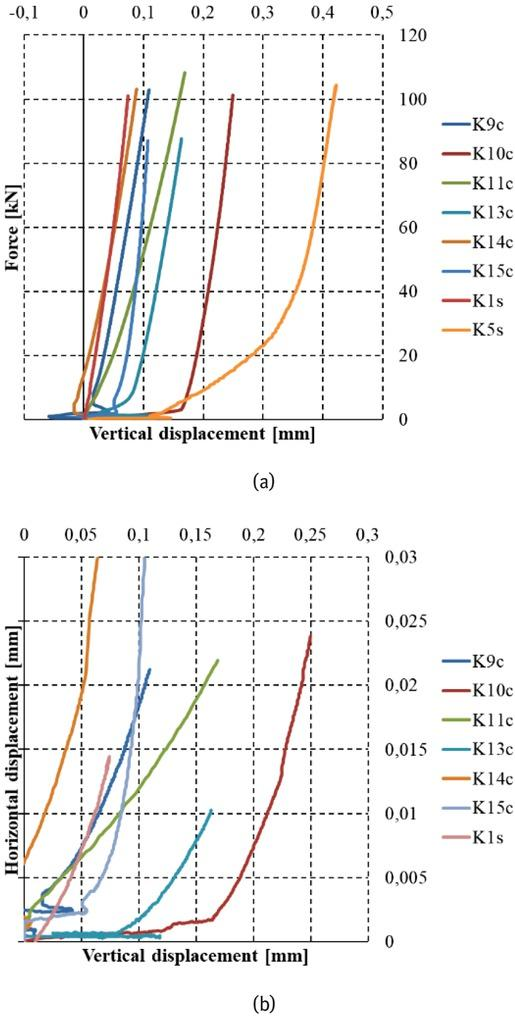Results of the compressive tests: a) relation between the force and vertical displacement, b) relation between horizontal and vertical displacement.

Poisson’s ratio was estimated on the basis of the curves showing the dependence of transverse displacements of the sample (measured with the extensometer) on the vertical displacements recorded by the displacement sensor (Fig. 5b) The calculated Poisson’s ratio varied from 0.1199 to 0.2909. Mean value was 0.2025 with standard deviation 0.0694 (34% of the mean value). The compressive strength was obtained from the standard method as the ratio of the destructive force to the area of horizontal cross-section of the sample. The mean value was 187.23 MPa with standard deviation 18.46 MPa (~10% of the mean value).

Beam bending test

The authors have performed three point bending test on notched beams, of the discussed material, to calculate the stress intensity factor in mode I and then the critical strain energy release rate in mode I.

Critical stress intensity factor is a material characteristic. It specifies the amount of stress concentration at the crack tip. There are three main modes of cracks. In the case of the problem described in this article, the most appropriate mode is mode I, which occurs when the crack opening caused by tensile force is perpendicular to the crack.

Several laboratory methods for testing the rate of crack energy release are used (van Mier, 1996). In the presented research, a three-point bending test was used, this test is performed on the samples notched in the middle of the span. Fig. 6 shows the static scheme and geometry of the samples, and Fig. 7 shows the photo taken during the laboratory test.

There are several methods for calculating the stress intensity factor in mode I from the results obtained from the described test (Bower, 2010; Elices, Guinea, Gómez, Planas, & Gomez, 2002). The authors of this article used the equation according to ASTM described in (Brown & Srawley, 1966):

$Kκ=3⋅Pc⋅I2⋅h2⋅b⋅π⋅a⋅(1.090−1.735ah+8.20(ah)2−14.18(ah)3+14.57(ah)4)$$${{K}_{\kappa }}=\frac{3\cdot {{P}_{c}}\cdot I}{2\cdot {{h}^{2}}\cdot b}\cdot \sqrt{\pi \cdot a}\cdot \left( 1.090-1.735\frac{a}{h}+8.20{{\left( \frac{a}{h} \right)}^{2}}-14.18{{\left( \frac{a}{h} \right)}^{3}}+14.57{{\left( \frac{a}{h} \right)}^{4}} \right)$$

Pc used in this equation is the force that initiates the crack in this bending test, a is the length of the notch, h – the height of the beam, b – the width of the beam, l – length of the beam. Six samples were used for this test. The calculated stress intensity factor is 69.184 N/mm3/2, which is close to factors obtained by other researchers for similar materials (Hasanpour & Choupani, 2008). The standard deviation was 5.500 N/ mm3/2 (8% of the mean value).

The critical strain energy release rate in mode I was calculated from the equation (Elices et al., 2002):

$GIc=KIc2E$$${{G}_{Ic}}=\frac{K_{Ic}^{2}}{E}$$

Its value is 0.306 N/mm.

Quasi-Brazilian test

The authors also made a quasi-Brazilian test on cubes. Typically, tests of traction during splitting are performed on cylindrical samples, but they are hard to obtain from such material as analyzed here. So, the Abaqus system was used to find the field of stresses and then the tensile strength for cubic samples. The tensile strength was calculated thanks to the results presented in the previous authors paper (Gontarz & Podgórski, 2016). Authors used the Ottosen-Podgórski criterion, which allows to calculate the tensile strength based on the equation:

$ft=σmaxρ$$${{f}_{t}}=\frac{{{\sigma }_{\max }}}{\rho }$$

where σmax is the tensile stress in the center of the sample, obtained from own numerical analyzes, ρ is the coefficient depending on the ratio of tensile and compressive stress and the chosen failure criterion for material in a complex stress state. For the chosen criterion and material, ρ ≈ 0.969, which shows that the tensile strength is slightly greater than the critical tensile stress. For the four examined samples, the tensile strength is 12.89 MPa with standard deviation 3.72 MPa (29% of the mean value).

In Fig. 8 samples after the exemplary tests are shown. The heterogeneity of the examined material can be seen.

Computer Simulations

The above material parameters were used to model the test in Abaqus for the 9 cm anchoring. The authors used the X-FEM method of crack propagation. Extended Finite Element Method is a method of simulating a fracture in the Finite Element Method, which is independent of the mesh. Modification of the shape function of element allows the finite element to be separated anywhere (Mohammadi, 2008), so the mesh doesn’t need to be fine. Crack initiation refers to the beginning of the degradation of the cohesive response in the enriched element. The degradation process begins when stresses or strains meet certain crack initiation criteria. Crack initiation criteria are available based on the following built-in Abaqus/Standard models: the maximum principal stress criterion, the maximum principal strain criterion, the maximum nominal stress criterion, the maximum nominal strain criterion, the quadratic traction-interaction criterion, and the quadratic separation-interaction criterion. An additional crack is introduced or crack length of an existing gap is carried on after an equilibrium increment, when the crack propagation criterion f = 1.0 (Abaqus V 6.14.2, 2014). In the above example, the simplest criterion for crack initiation is chosen – the maximum principal stress damage, which occurs when the tensile stress exceeds the value of the tensile strength. The damage evolution can be determined based on fracture energy or displacement at failure. In

this simulation, energy is the most-suited choice, because the critical strain energy release rate was calculated from laboratory tests (GIc = 0.306 N/mm).

As it was described before, 7 methods of the pull-out force application were selected:

without anchor, locked horizontal displacement,

without anchor, free horizontal displacement,

with anchor, friction coefficient μ = 0.01,

with anchor, friction coefficient μ = 0.1,

with anchor, friction coefficient μ = 0.2,

with anchor, friction coefficient μ = 0.5,

with anchor, friction coefficient μ = 1.0.

Given below are some exemplary figures from simulations in Abaqus. In Fig. 9, one of the models before the load application is shown. The view of the damaged model from a sample simulation is shown in Fig. 10.

As it can be seen, the crack started to propagate as expected, but near the upper edge of the model, the crack began to distort and return. For various FEA system settings and different meshes, it was not possible to cause the crack to go through to the end. This is related to the limitations of X-FEM in Abaqus, probably due to a complex stress state in which the procedures implemented in system are not precise enough to correctly predict the direction of the crack propagation. Analyzing the results of the simulation, we can observe that the force applied to the anchor increases until the crack reaches the size equal approximately to the anchorage depth. At this point, the gap propagates with decreasing value of force. Fig. 11 shows the relationship between the crack range and the pull-out force that was obtained during the FEM simulation. As can be seen, there were significant differences in the values of the critical forces obtained, which were derived from the method of load simulation and anchor-rock contact. The maximum force varies from about 100 kN to 220 kN. Given below are all the maximum force values for different methods that have been achieved: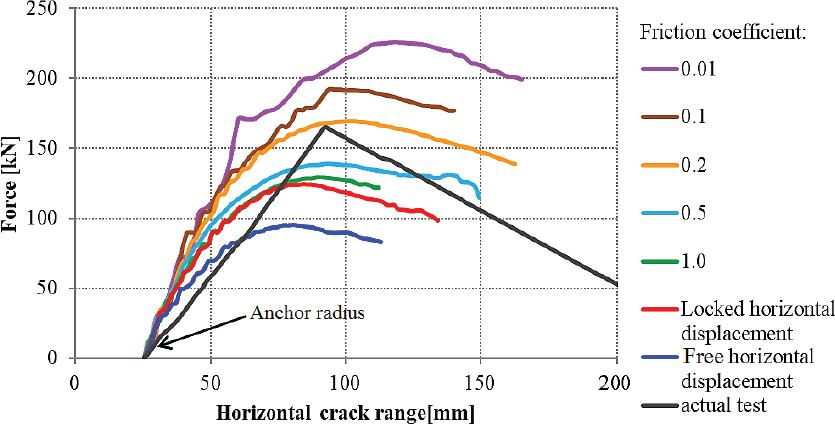Graph of force dependence on the horizontal range of the crack.

locked horizontal displacement: 124.52 kN,

with free horizontal displacement: 95.31 kN,

friction coefficient μ = 0.01: 225.90 kN,

friction coefficient μ = 0.1: 190.67 kN,

friction coefficient μ = 0.2: 169.40 kN,

friction coefficient μ = 1.0: 129.25 kN,

In Fig. 12, crack paths for different methods are shown. Here, the results for simulations without anchor are so unnatural that they can’t be taken into account further. Nevertheless the results for simulations with anchor are also very different to each other. The maximum force is also obtained in different locations, with different crack lengths. It means that the model and the friction coefficient should be chosen very carefully, but this is a difficult task due to the fact that the material crushes in contact with the anchor. As it will be proved in the next section, the shape closest to reality is the one for the friction coefficient μ = 0.2.

It can be seen that the path of the crack at the very end behaves unnaturally in all the models. It should also be noted that the line for actual test is approximate because it is physically impossible to acquire such information during the test. This test is described in the next section.

The main conclusion from the above analysis is that anchor presence should not be neglected in the analysis; however, different values of the friction coefficients have a very significant influence on the result, both on the maximum force and the crack path.

Pull-Out Test on Actual Rock

Tests in the quarry were also made on the same stone and for the same depth of the anchor (Fig. 13). For three successful tests performed on a sandstone, the average pulling-out force is 162 kN (Jonak et al., 2019). Inspection of the damaged rock in Fig. 14 allowed to state that the shape of the broken fragment is similar to the one in the computer simulation, especially for the simulation with the anchor-rock friction coefficient μ ≈ 0.2.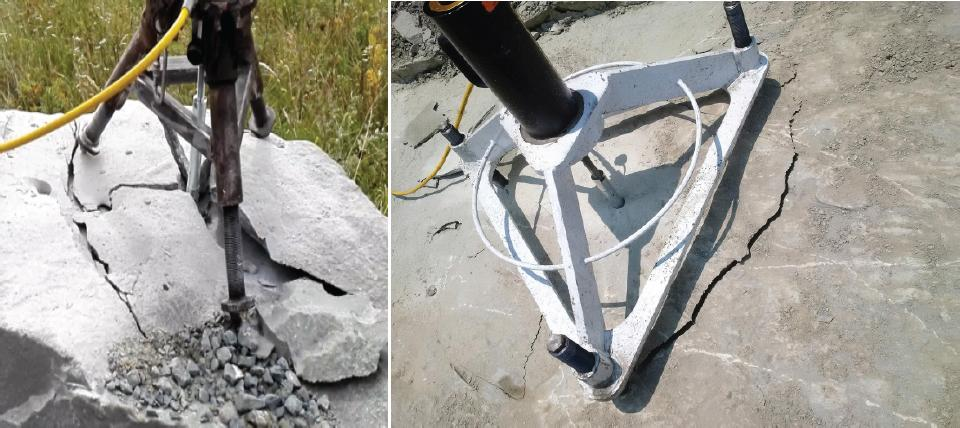Pull-out test performed on actual rock (Jonak et al., 2019).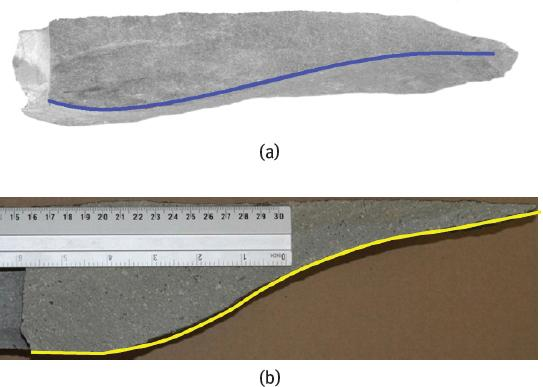A cross-section of the actual pull-out sample: a) Sandstone, b) Porphyry

Examining one of the pulled-out cone in Fig. 15, it is also possible to explain the incorrect crack behavior in computer simulations. It is evident that the width of the pulled-out fragment is different on the circumference. For most tests, the rock breaks perpendicular to the surface when the gap approaches it at a distance of 1¸2 cm. Probably in simulations, there should also be such a crack, but the Abaqus system has difficulty in simulating the crack forking. The best obtained result is for simulation with anchor and friction coefficient between the anchor and the rock μ = 0.2, both in terms of the maximum obtained force and the shape of the crack. However, it is not known if this coefficient is correct, especially since in fact this coefficient changes due to the change of the rock structure in the contact zone, high pressure in this area causes crushing of the rock.

Determining the Direction of the Crack Propagation

For the reason that Abaqus FEA system has difficulties to correctly lead the crack, authors are planning to implement their own “Abaqus User Subroutine”, which will use its own crack propagation criterium. Abaqus determines the crack direction based on the direction of maximum principal stress occurring in the finite element in which the crack occurs. In the proposed method, finding the direction of the crack propagation requires calculation of the “failure parameter” μ (material effort ratio): its value is calculated as a ratio rσ/rf of vectors modules in the stresses space (Podgórski, 2017), as it can be seen in Fig. 16.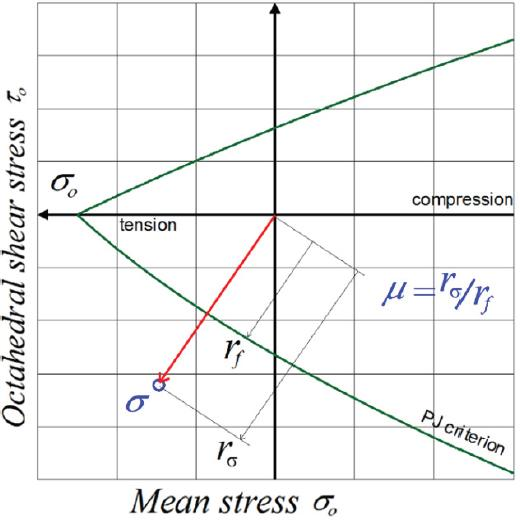Definition of the material effort ratio μ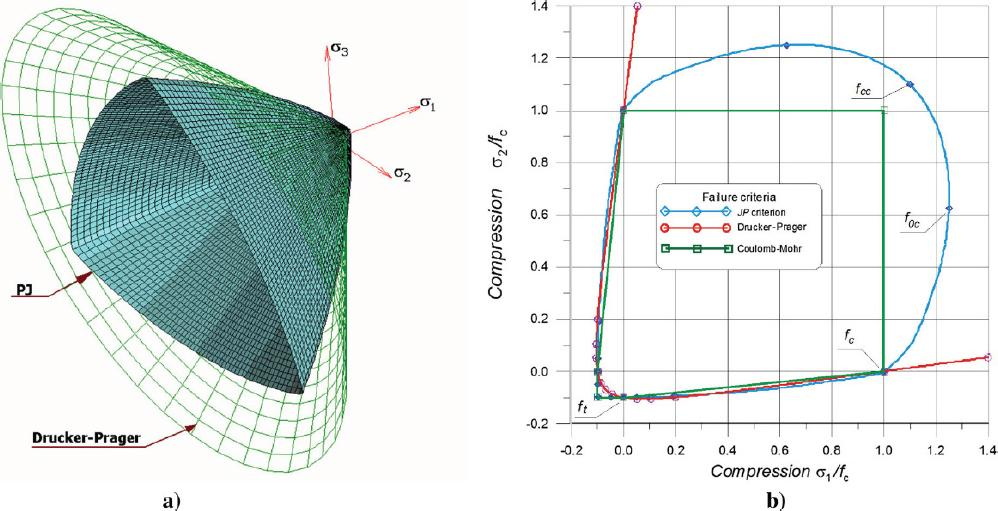a) The limit surface associated with author Ottosen-Podgórski (PJ) criterion compared with Drucker-Prager cone, b) Coulomb-Mohr, Drucker-Prager and PJ criteria at 2D stress state.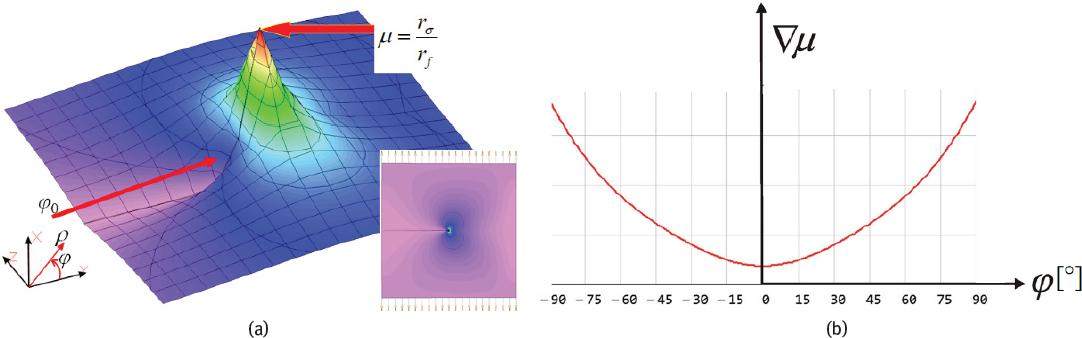a) Values of the material effort ratio μ near the crack tip, b) the exemplary gradient-crack direction dependency.

The rσ parameter is the distance in the stress space between point 0, and the point describing the current stress state, rf is the distance in the stress space between the point 0 and the point on the failure criterion envelope:

$rσ=τ02+σ02,rf=τf2+σf2$$${{r}_{\sigma }}=\sqrt{\tau _{0}^{2}+\sigma _{0}^{2}},\,\,\,\,\,\,{{r}_{f}}=\sqrt{\tau _{f}^{2}+\sigma _{f}^{2}}$$

where τ0 and σ0 are stress invariants, which can be calculated for the chosen failure criterion. Authors have chosen the Ottosen-Podgórski (PJ) criterion (Podgórski, 1984), (Podgórski, 1985), which can be written in the form:

$σ0−C0+C1PJτ0+C2τ02=0$$${{\sigma }_{0}}-{{C}_{0}}+{{C}_{1}}P\left( J \right){{\tau }_{0}}+{{C}_{2}}\tau _{0}^{2}=0$$

Where P(J) is a function describing the shape of limit surface in deviatoric plane and α, β, C0, C1, C2 are material constants. The limit surface for this criterion is shown in Fig. 17.

The failure parameter μ is calculated for a small area around the crack tip. It is calculated for any applied force, and when it is greater than 1, then the failure occurs. Applied force then is reduced proportionally to parameter μ, to receive the force that initiates the crack. The next important aspect is the crack propagation direction. The appropriate direction φ of the crack propagation is chosen, for which the maximum value of the failure parameter gradient is achieved:

$∇μφ0=∂μ∂r|φ=φ0→min$$$\nabla \mu \left( {{\varphi }_{0}} \right)=\frac{\partial \mu }{\partial r}{{|}_{\varphi ={{\varphi }_{0}}}}\to \text{min}$$

The algorithm for own Abaqus User Subroutine is simple in assumptions. When the crack reaches a specific finite element the gradient function is calculated for angle φ from -90° to 90°. The angle for minimum gradient is chosen and the crack is carried out at this angle to the next finite element. The shape function of the element with the crack is enriched as in typical X-FEM analysis.

The authors decided to pre-check the operation of this algorithm in one selected point of the calculation. Element near the beginning of the crack was chosen, which is the area where the crack path appointed by Abaqus was correct. For this purpose, stresses σ11, σ22, σ33 and τ12 were read in several distances from the crack tip (from 0.4 mm to 1.1 mm, where the average size of finite element is 1 mm near the crack path). Then, these stresses were transformed into the σ0 - τ0 plane. The paths after which the stresses were read are presented in Fig. 19 (red arcs). Then σf and τf were calculated from equation and proportion s0/t0 = sf /τf for every angle from -90° to 90° and for each circumference of the arc. Then the material effort was calculated from the following equation: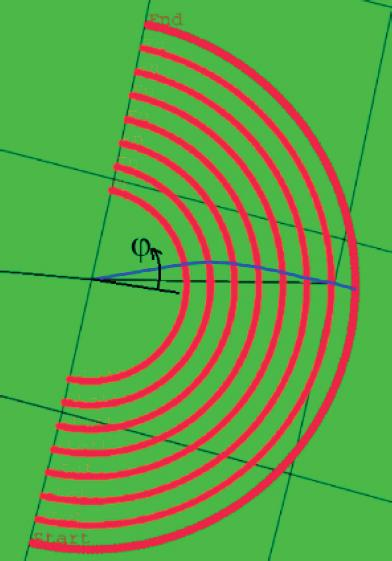Predicted crack path found with own method.$μ=σ02+τ02σf2+τf2$$$\mu =\frac{\sqrt{\sigma _{0}^{2}+\tau _{0}^{2}}}{\sigma _{f}^{2}+\tau _{f}^{2}}$$

The values of material effort ratio are presented in Fig. 20. Instead of finding the gradient of material effort, the authors stated in this case that the crack will go in the direction where the material effort is minimum. It allowed to find the crack path within the range of the assumed arches (blue line in Fig. 19). It is worth noticing that in Fig. 20, there are some vertical shifts on the curves. This is due to the fact that there are finite element edges. Stresses in the two adjacent finite elements are different, while inside the element stress, values are interpolated.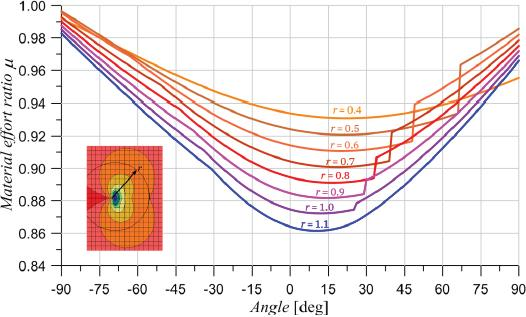Values of material effort ratio for different angles and different distance from the crack tip.

Returning to Fig. 19, X-FEM method does not assume cracking along the curve, but only in a straight line. Therefore, the only important thing here is the point of intersection of the predicted crack curve with the edge of the tested finite element, and this is the point where the crack tip should appear in the next increment of the simulation. Initial analysis showed promising results, because this point coincides with the point determined by the Abaqus program. Next step of these considerations would be checking the same procedure in further increments of the calculation, that is, where Abaqus began to give wrong directions of the crack. Then, if the method turned out to be correct, the next step would be programming the discussed algorithm as own Abaqus User Subroutine.

Summary

As it was stated before, the results without an anchor are incorrect in relation to the models with an anchor. Computer simulations can’t be performed to the total breakage of the rock fragment, because before the end, the crack begins to behave inconsistently to the test in reality. Fortunately, the maximum force was obtained before the occurrence of this phenomenon, which means that even if this phenomenon continues to occur, it is

possible to determine the maximum force. Authors are planning to implement own “Abaqus User Subroutine” in Abaqus system with own crack propagation criterion, and hopefully, it will allow to correctly lead the crack as in a real rock.

Abaqus V 6.14.2 User’s Manual. (2014). Retrieved from https://www.sharcnet.ca/Software/Abaqus/6.14.2/v6.14/books/usb/default.htm?startat=pt04ch10s07at36.htmlAbaqus V 6.14.2 User’s Manual2014Retrieved fromhttps://www.sharcnet.ca/Software/Abaqus/6.14.2/v6.14/books/usb/default.htm?startat=pt04ch10s07at36.htmlSearch in Google Scholar

Bower, A. F. (2010). Applied mechanics of solids CRC Press.BowerA. F.2010Applied mechanics of solidsCRC Press10.1201/9781439802489Search in Google Scholar

Brencich, A. (2015). A post-installed insert for pull-out tests on concrete up to 70 MPa. Construction and Building Materials 95, 788–801. https://doi.org/10.1016/J.CONBUILDMAT.2015.07.055BrencichA.2015A post-installed insert for pull-out tests on concrete up to 70 MPaConstruction and Building Materials95788801https://doi.org/10.1016/J.CONBUILDMAT.2015.07.05510.1016/j.conbuildmat.2015.07.055Search in Google Scholar

Brown, W. F., & Srawley, J. E. (1966). Plane Strain Crack Toughness Testing of High Strength Metallic Materials Philadelphia: ASTM International. https://doi.org/10.1520/STP410-EBBrownW. F.&SrawleyJ. E.1966Plane Strain Crack Toughness Testing of High Strength Metallic MaterialsPhiladelphiaASTM Internationalhttps://doi.org/10.1520/STP410-EB10.1520/STP44663SSearch in Google Scholar

Contrafatto, L., & Cosenza, R. (2014). Behaviour of post-installed adhesive anchors in natural stone. Construction and Building Materials 68, 355–369. https://doi.org/10.1016/j.conbuildmat.2014.05.099ContrafattoL.&CosenzaR.2014Behaviour of post-installed adhesive anchors in natural stoneConstruction and Building Materials68355369https://doi.org/10.1016/j.conbuildmat.2014.05.09910.1016/j.conbuildmat.2014.05.099Search in Google Scholar

Elices, M., Guinea, G. V. G., Gómez, J., Planas, J., & Gomez, J. (2002). The cohesive zone model: advantages, limitations and challenges. Engineering Fracture Mechanics 69(2), 137–163. https://doi.org/10.1016/S0013-7944(01)00083-2ElicesM.GuineaG. V. G.GómezJ.PlanasJ.&GomezJ.2002The cohesive zone model: advantages, limitations and challengesEngineering Fracture Mechanics692137163https://doi.org/10.1016/S0013-7944(01)00083-210.1016/S0013-7944(01)00083-2Search in Google Scholar

European Technical Assessment ETA-99/0009 of 06/01/2015 for Hilti HDA and HDA-R anchor. (n.d.).European Technical Assessment ETA-99/0009 of 06/01/2015 for Hilti HDA and HDA-R anchor. (n.d.)Search in Google Scholar

Gontarz, J., & Podgórski, J. (2016). Explanation of the mechanism of destruction of the cylindrical sample in the Brazilian test. In M. Kleiber, T. Burczyński, K. Wilde, J. Górski, K. Winkelmann, & Ł. Smakosz (Eds.), Advances in Mechanics : Theoretical, Computational and Interdisciplinary Issues (pp. 479–483). Gdańsk: Boca Raton.GontarzJ.&PodgórskiJ.2016Explanation of the mechanism of destruction of the cylindrical sample in the Brazilian testKleiberM.BurczyńskiT.WildeK.GórskiJ.WinkelmannK.&SmakoszŁ.(Eds.)Advances in Mechanics : Theoretical, Computational and Interdisciplinary Issues479483GdańskBoca Raton10.1201/b20057-102Search in Google Scholar

Hasanpour, R., & Choupani, N. (2008). Mixed-Mode Study of Rock Fracture Mechanics by using the Modified Arcan Specimen Test. International Journal of Geotechnical and Geological Engineering 2(5), 716–721.HasanpourR.&ChoupaniN.2008Mixed-Mode Study of Rock Fracture Mechanics by using the Modified Arcan Specimen TestInternational Journal of Geotechnical and Geological Engineering25716721Search in Google Scholar

Jonak, J., Kalita, M., Siegmund, M., & Podgórski, J. (2019). Raport NCN project RODEST nr 2015/19/B/ST10/02817JonakJ.KalitaM.SiegmundM.&PodgórskiJ.2019Raport NCN project RODEST nr 2015/19/B/ST10/02817Search in Google Scholar

Mier, J. G. M. van. (1996). Fracture processes of concrete CRC Press.MierJ. G. M. van.1996Fracture processes of concreteCRC PressSearch in Google Scholar

Mohammadi, S. (Soheil). (2008). Extended finite element method for fracture analysis of structures Blackwell Pub.MohammadiS2008Extended finite element method for fracture analysis of structuresBlackwell Pub10.1002/9780470697795Search in Google Scholar

Podgórski, J. (1984). Limit state condition and the dissipation funcion for isotropic materials. Arch. Mech 36(3), 323–342.PodgórskiJ.1984Limit state condition and the dissipation funcion for isotropic materialsArch. Mech363323342Search in Google Scholar

Podgórski, J. (1985). General Failure Criterion for Isotropic Media. Journ. Eng. Mech. ASCE 111(2), 188–201.PodgórskiJ.1985General Failure Criterion for Isotropic MediaJourn. Eng. Mech. ASCE111218820110.1061/(ASCE)0733-9399(1985)111:2(188)Search in Google Scholar

Podgórski, J. (2017). The criterion for determining the direction of crack propagation in a random pattern composites. Meccanica 52(8), 1923–1934. https://doi.org/10.1007 /s11012-016-0523-yPodgórskiJ.2017The criterion for determining the direction of crack propagation in a random pattern compositesMeccanica52819231934https://doi.org/10.1007/s11012-016-0523-y10.1007/s11012-016-0523-ySearch in Google Scholar

Wang, D., Wu, D., Ouyang, C., He, S., & Sun, X. (2017). Simulation analysis of large-diameter post-installed anchors in concrete. Construction and Building Materials 143, 558–565. https://doi.org/10.1016/j.conbuildmat.2017.03.149WangD.WuD.OuyangC.HeS.&SunX.2017Simulation analysis of large-diameter post-installed anchors in concreteConstruction and Building Materials143558565https://doi.org/10.1016/j.conbuildmat.2017.03.14910.1016/j.conbuildmat.2017.03.149Search in Google Scholar

• #### Resonance of a structure with soil elastic waves released in non-linear hysteretic soil upon unloading

Artículos recomendados de Trend MD